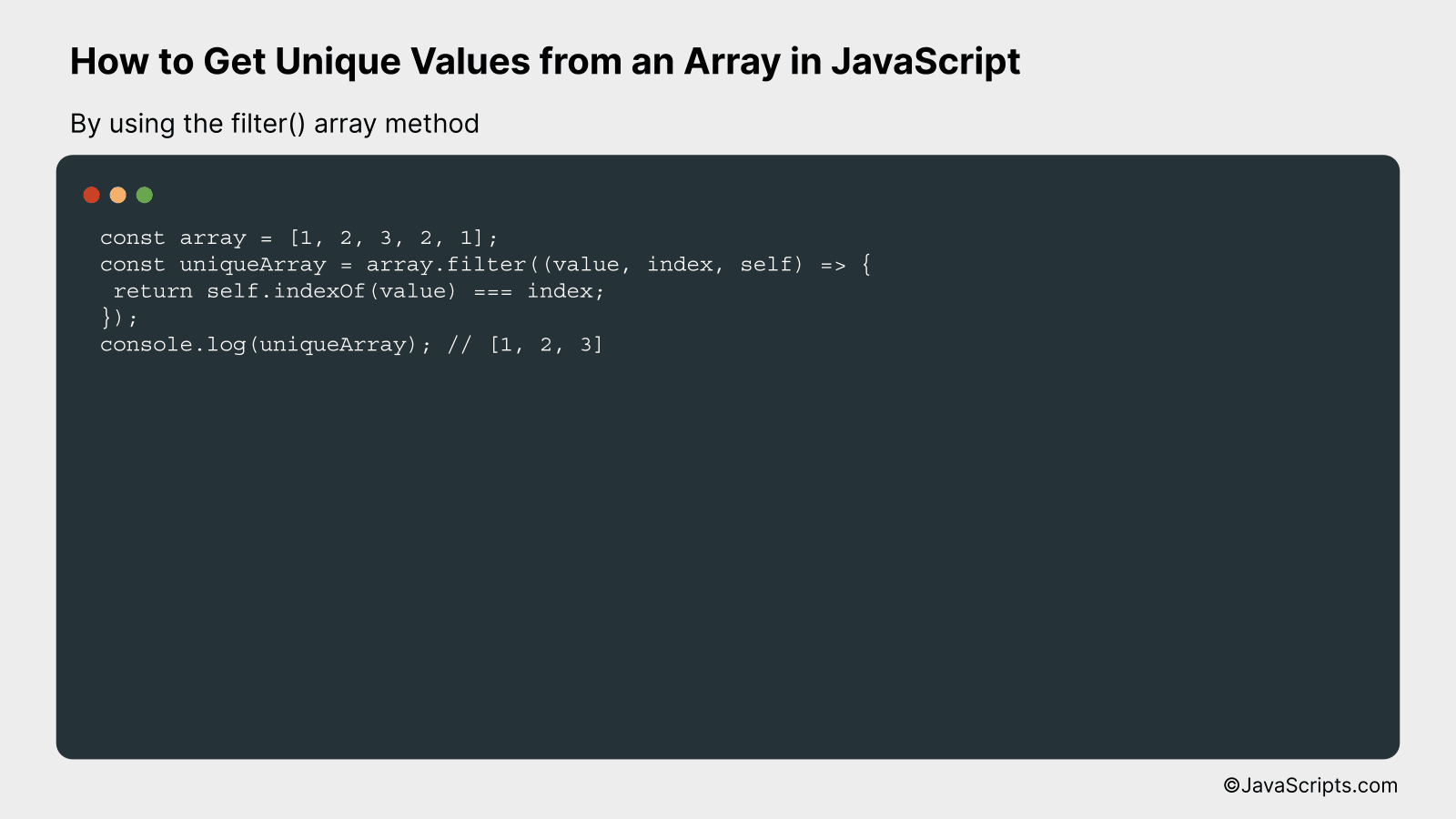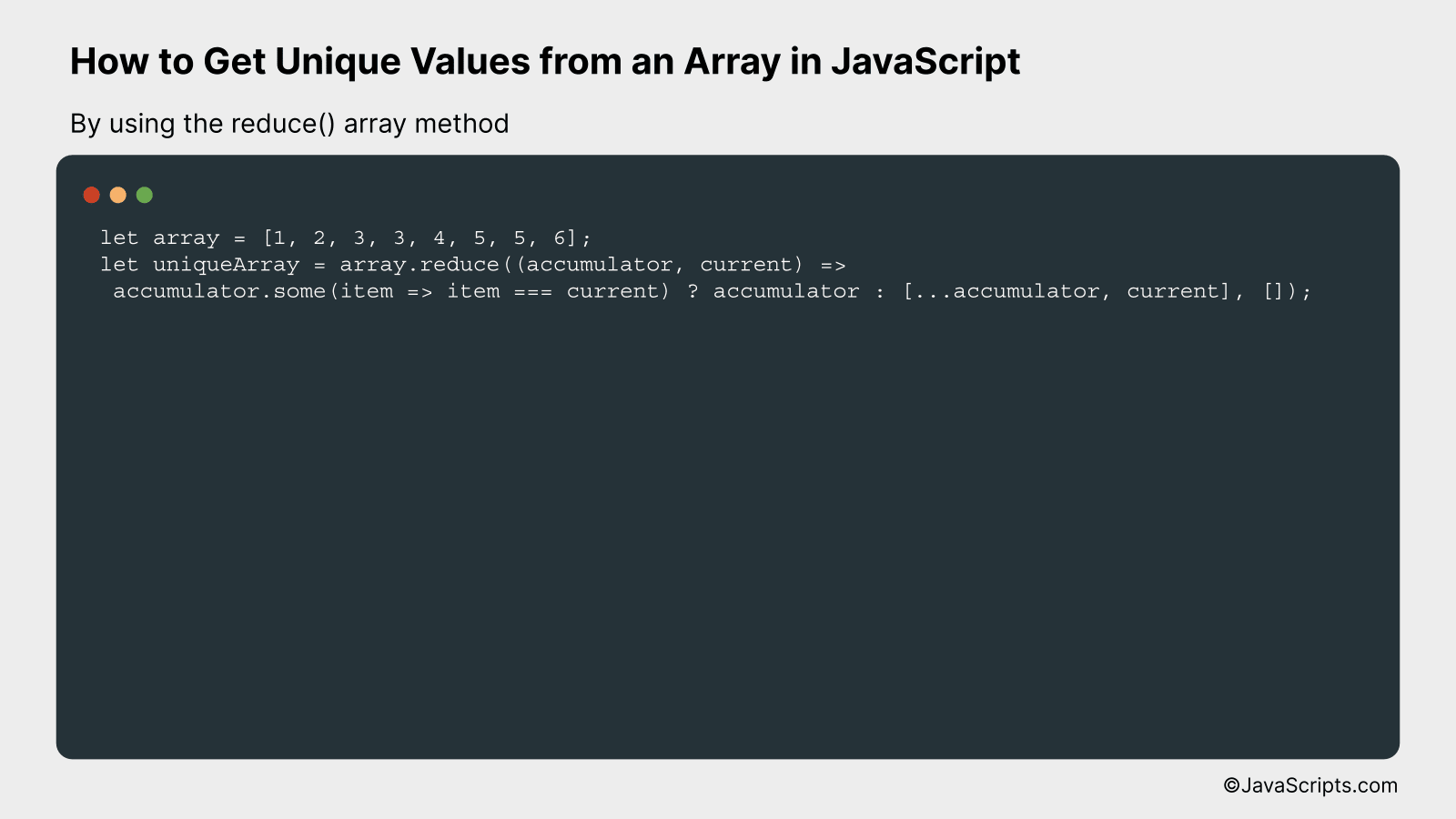# How to Get Unique Values from an Array in JavaScript

Harnessing the power of JavaScript to manipulate arrays can significantly streamline your coding efforts. If you’ve ever grappled with the challenge of extracting unique values from an array, then you’ve come to the right place.

You and I will go on a journey to uncover simple yet effective methods to achieve this. We’ll unravel the magic of JavaScript, making it work for us, not against us. Trust me, it’s easier than you think!

In this post, we will be looking at the following 3 ways to get unique values from an array in JavaScript:

• By using the Set object
• By using the filter() array method
• By using the reduce() array method

Let’s explore each…

## #1 – By using the Set objectThe Set object in JavaScript allows you to store unique values of any type. In this example, we’ll use the Set object to extract the unique values from an array in a single line of code.

``````
let array = [1, 2, 3, 2, 1];
let uniqueArray = [...new Set(array)];
console.log(uniqueArray); // Output: [1, 2, 3]
``````

### How it works

In this example, the Set object is used to store the unique values from the array. We then use the spread operator (…) to convert the Set back into an array. This results in an array that only contains the unique values.

• The input array is defined with some duplicate values: `let array = [1, 2, 3, 2, 1];`
• A new Set object is created with the array as an argument. The Set object automatically removes any duplicate values: `new Set(array)`
• The spread operator (…) is used to create a new array from the Set object: `[...new Set(array)]`
• This new array is stored in the variable uniqueArray: `let uniqueArray = [...new Set(array)];`
• Finally, uniqueArray is logged to the console and the output is the array with unique values: `console.log(uniqueArray); // Output: [1, 2, 3]`

## #2 – By using the filter() array methodThe filter() method in JavaScript is used to create a new array with all elements that pass a condition implemented by the provided function. To get unique values from an array using this method, we’ll compare the index of the current item to the first occurrence of that item in the array and only keep the items where these two indexes are the same. Here’s how you can do it:

``````
const array = [1, 2, 3, 2, 1];
const uniqueArray = array.filter((value, index, self) => {
return self.indexOf(value) === index;
});
console.log(uniqueArray); // [1, 2, 3]
``````

### How it works

The filter() method is applied on the array. For every element in the array, it checks if the first occurrence of the current value is at the same position as the current index. If so, it includes that value in the new array, thereby ensuring that only unique values are included.

• The filter() function loops through each value in the array.
• For each value, it invokes a function where it takes three arguments: the current value, the current index, and the original array.
• The condition inside the filter function checks if the index of the current value is the same as the current index. This condition will only be true for the first occurrence of a unique value in the array, thus eliminating duplicates.
• If the condition is true, the value is added to the new ‘uniqueArray’.
• Finally, the uniqueArray is logged on the console, which will contain only the unique values from the original array.

## #3 – By using the reduce() array methodThis method will create a new array with unique elements by using the JavaScript reduce() method in combination with the some() method. It works by iteratively checking if each array element exists in the accumulator array and, if not, adding it.

``````
let array = [1, 2, 3, 3, 4, 5, 5, 6];
let uniqueArray = array.reduce((accumulator, current) =>
accumulator.some(item => item === current) ? accumulator : [...accumulator, current], []);
``````

### How it works

The JavaScript reduce() method is used to reduce the array to a single value, which in this case, is an array of unique values. It does so by iterating through each item in the source array and applying a reducer function to an accumulated value and the current item.

• The reduce() function takes two parameters – the reducer function and the initial value of the accumulator. Here, the initial value of the accumulator is an empty array.
• The reducer function is called for each item in the array. It also takes two parameters – the accumulator and the current item.
• Inside the reducer function, the some() method is used to check if the current item already exists in the accumulator array.
• If the current item exists in the accumulator array (some() returns true), the accumulator is returned without any changes. This effectively ignores the current item.
• If the current item does not exist in the accumulator array (some() returns false), a new array is created by combining the accumulator array and the current item, thus adding the current item to the unique array.
• The reduce() method finally returns the accumulator array which now contains only unique items.

Related:

In conclusion, JavaScript provides us with various methods to extract unique values from an array effortlessly. The methods we’ve discussed, such as ‘Set’ and ‘Filter’, are simple yet powerful tools to refine our data.

Applying these techniques, we can improve data quality and optimize our code. Remember, understanding and utilizing such features of JavaScript can make our coding journey much more productive and enjoyable.